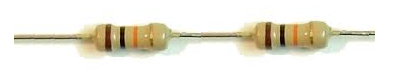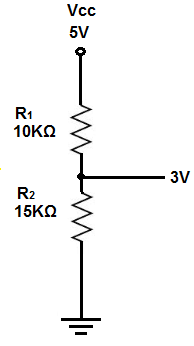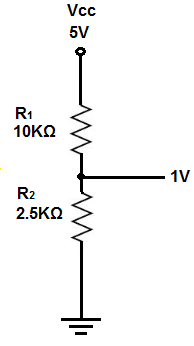﻿ How to Reduce Voltage with Resistors ﻿# How to Reduce Voltage with ResistorsSay if you have a main power supply that is supplying a voltage that is much higher than you may need for a particular part of a circuit, such as for a chip in the circuit. Maybe the circuit is run off of a 9-volt battery but the chip in the circuit only needs 3V.

How can this voltage be reduced?

It turns out, there are many ways of doing so, and one of the easiest and cheapest ways of doing so is simply using resistors.

With resistors, we can form a voltage divider circuit, so that we can obtain any voltage we want.

To know how to reduce voltage, you simply just have to understand how the math works for voltage divider circuits, and we will go over this below. Through this technique, you can take any voltage and lower it to any level you want.

### How to Reduce Voltage in Half

To reduce voltage in half, we simply form a voltage divider circuit between 2 resistors of equal value (for example, 2 10KΩ) resistors.To divide voltage in half, all you must do is place any 2 resistors of equal value in series and then place a jumper wire in between the resistors. At this point where the jumper wire is placed, the voltage will be one-half the value of the voltage supplying the circuit.

The 5V is now 2.5V. VCC is split in half.

### How to Reduce Voltage to Any Value

You may not always want half the voltage, but you can get the voltage to any level you want choosing the appropriate resistor values for a voltage divider circuit.

Maybe your circuit is running off of 5V, yet you want only 3V to feed into a particular circuit element. How can you get the 5 volts into 3V?

And you can do so with the correct resistor values in a voltage divider circuit.

How do you calculate it?

The formula for calculating the voltage that falls across the R2 resistor is shown below.But this formula, in its original form, does not help us in finding the resistor R2 we need for getting a particular voltage.

Rearranging this formula and solving for R2 yields the formula below.This is the formula we can use for choosing the resistor value we need to get any voltage we want.

So back to our circuit, if we have 5 volts and want 3V from it, we use the formula above. If we use a 10KΩ as our R1 resistor, plugging in the values, we get R2= (V)(R1)/(VIN - V)= (3V)(10KΩ)/(5V - 3V)= 15KΩ. So we can use a 15KΩ resistor as our R2 resistor with the R1 resistor being 10KΩ.Let's do one more example.

Say if you have the same circuit above with 5V but only want 1V. If we use a 10KΩ as our R1 resistor, plugging in the values, we get R2= (V)(R1)/(VIN - V)= (1V)(10KΩ)/(5V - 1V)= 2.5KΩ. So we can use a 2.5KΩ resistor as our R2 resistor with the R1 resistor being 10KΩ.So it's easy to see now how any voltage can be obtained with a resistor voltage divider circuit.

To check calculations for voltage dividers, see the Voltage Divider Calculator. This way, you can check calculations you've made to see if they are correct and accurate. This voltage divider calculator computes voltage division from 2 resistors to 10 resistors in a voltage divider circuit.

Related Resources